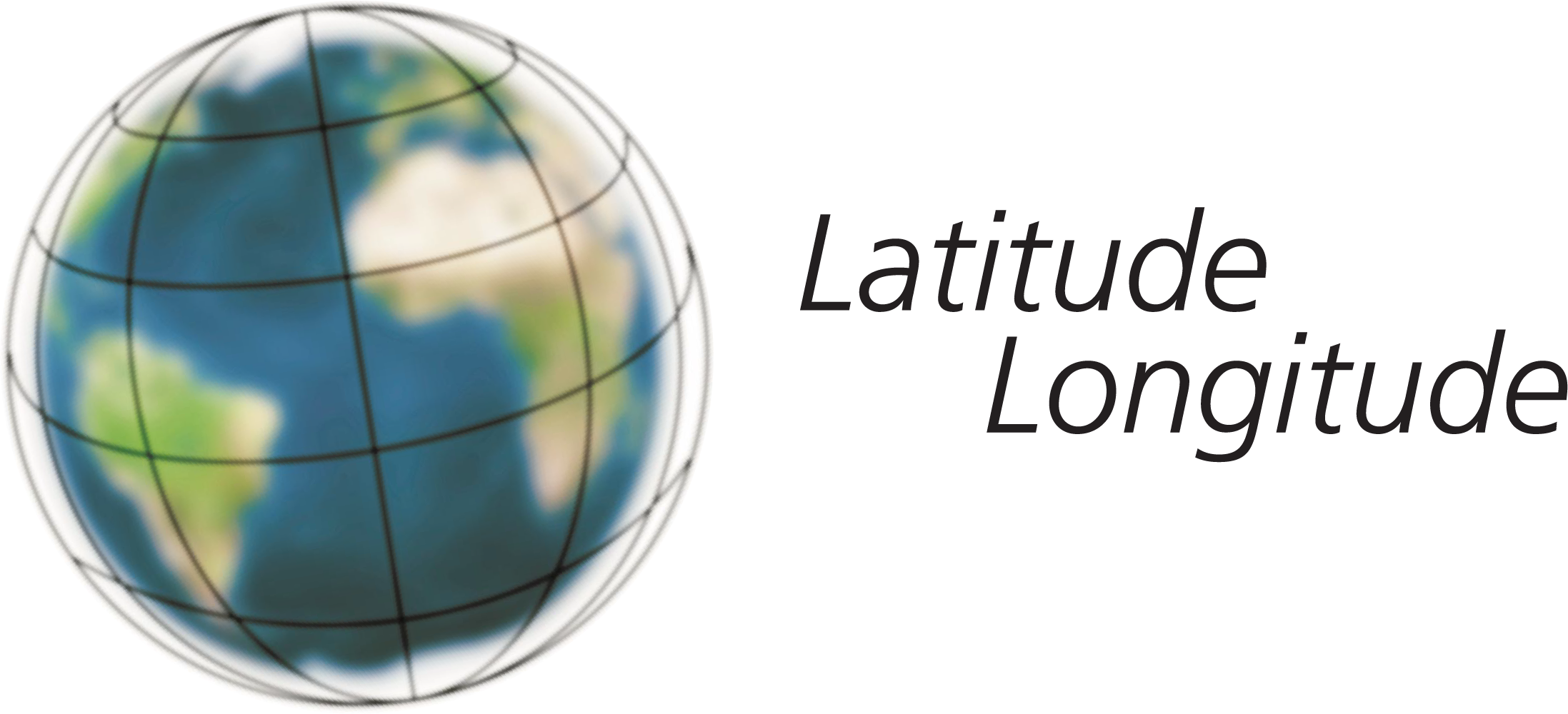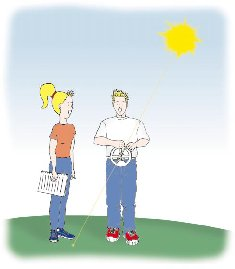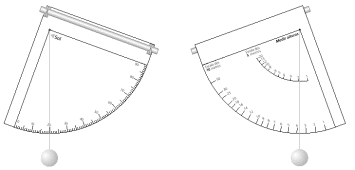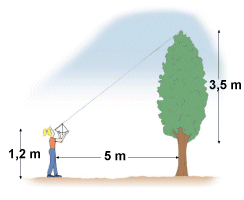## THE ASTROLABE AND THE QUADRANT

Notebook "Where are you?"An astrolabe is an instrument used to measure angles. About 500 years ago, Portuguese navigators who discovered the islands of the Azores, Cape Verde and Brazil used the astrolabe so that they would not get lost at sea. Using the astrolabe they were able to calculate their position on earth by measuring the angle of the Sun above the horizon.The Astrolabe that the Portuguese navigators used on board ship to calculate the height of the Sun throughout the day.Using the astrolabe to measure the angle of the Sun above the horizon.

You can find out the height of your house, your school or trees in a park with the aid of an astrolabe. This can also be done with a quadrant, which is like the fourth part of an astrolabe.

### Experiment

Cut out the card quadrant and the four black dots on the flaps.

Fold the flaps over and insert a straw through the holes.

Use a needle to put a thread through the hole on the quadrant. Tie the two ends so that the quadrant hangs on the loop.

Tie a weight onto the thread so that it hangs down.### Be very careful when you are using your quadrant - you should never look at the Sun through the straw because you could damage your eyes.

We can also use the quadrant to measure the height of a tree in a park.

On one side of your quadrant, there is a scale in degrees just like the protractor. This scale measures a deviation or an angle above the horizon. On the other side, the scale gives you the height of an object.

### To find out the height of a tree, follow these instructions:

Choose the tree you want to measure. Stand five metres - five large steps - away from it in a straight line in any direction and then turn to face the tree.

Hold up your quadrant and look at the top of the tree through the straw. The thread shows a number on the five metre scale on the quadrant. This figure is the height of the tree from your eye level.

To find out the height of the tree, measure the distance from your eyes to the ground and add this to the number you read on the quadrant. E.g. if you read 3.5m. on the quadrant and you are 1.20m. tall, the height of the tree is 3.5 + 1.20 = 4.70m. On the other side of the quadrant, you can see the angle from which you are looking at the tree. The higher the tree, the greater the angle.

You can repeat this experiment with higher houses from a distance of 10 metres. In this case you must use the ten metre scale on your quadrant to find out the height.